# Intermediate Algebra Tutorial 32

Intermediate Algebra
Tutorial 32: Multiplying and Dividing Rational Expressions

WTAMU > Virtual Math Lab > Intermediate AlgebraLearning Objectives

After completing this tutorial, you should be able to:
1. Find the domain of a rational function.
2. Simplify a rational expression.
3. Multiply and divide rational expressions.Introduction

Do you ever feel like running and hiding when you see a fraction? If so, you are not alone.  But don't fear!  Help is here!  Hey, that rhymes.  Anyway, over the next several tutorials we will be showing you several aspects of rational expressions (fractions).   In this section we will be simplifying them.  Again, we will be putting your knowledge of factoring to the test.  Factoring plays a big part of simplifying these rational expressions. We will also look at multiplying and dividing them.  I think you are ready to tackle these rational expressions.Tutorial

Rational Expression or Function

A rational expression or function is
one that can be written in the form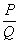where P and Q are polynomials and Q does not equal 0.

An example of a rational expression is :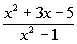Domain of a Rational Function

Recall from Tutorial 13: Introduction to Functions that the domain of a function is the set of all input values to which the rule applies.

With rational functions, we need to watch out for values that cause our denominator to be 0.  If our denominator is 0, then we have an undefined value.

So, when looking for the domain of a given rational function, we use a back door approach.  We find the values that we cannot use, which would be values that make the denominator 0.Example 1:   Find the domain of the function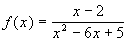.

Our restriction is that the denominator of a fraction can never be equal to 0.

So to find our domain, we want to set the denominator “not equal” to 0 to restrict those values.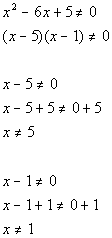*Restrict values that cause den. to be 0

*"Solve" for x

*"Solve" for x

Our domain is all real numbers except 5 and 1 because they both make the denominator equal to 0, which would not give us a real number answer for our function.

Fundamental Principle of
Rational Expressions

For any rational expression, and
any polynomial R, where ,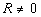, then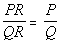In other words, if you multiply the EXACT SAME thing to the numerator and denominator, then you have an equivalent rational expression.

This will come in handy when we simplify rational expressions, which is coming up next.

Simplifying (or reducing) a
Rational Expression

Step 1: Factor the numerator and the denominator.

If you need a review on factoring, you can go to any or all of the following tutorials:

Tutorial 29: Factoring Special Products

Step 2: Divide out all common factors that the numerator and the denominator have.Example 2:  Write the rational expression in lowest terms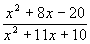.

Step 1: Factor the numerator and the denominator

AND

Step 2: Divide out all common factors that the numerator and the denominator have.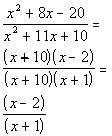*Factor the num. and den.

*Divide out the common factor of (x + 10)

Multiplying Rational Expressions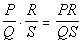Q and S do not equal 0.

Step 1: Factor both the numerator and the denominator.

If you need a review on factoring, you can go to any or all of the following tutorials:

Tutorial 29: Factoring Special Products

Step 2: Write as one fraction.

Write it as a product of the factors of the numerators over the product of the factors of the denominators.  DO NOT multiply anything out at this point.

Step 3: Simplify the rational expression.Example 3:   Multiply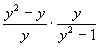.

Step 1: Factor both the numerator and the denominator

AND

Step 2: Write as one fraction.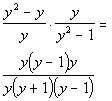*Factor the num. and den.

Step 3: Simplify the rational expression.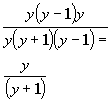*Divide out the common factors of y and (y - 1)

Dividing Rational Expressions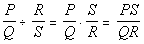where Q, S, and R do not equal 0.

Step 1: Write as multiplication of the reciprocal.

Step 2: Multiply the rational expressions as shown above.Example 4:   Divide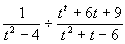.

Step 1: Write as multiplication of the reciprocal

AND

Step 2: Multiply the rational expressions as shown above.*Rewrite as mult. of reciprocal

*Factor the num. and den.

*Div. out the common factors of
(t + 3) and (t - 2)Example 5:   Multiply and divide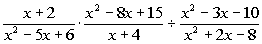.

Since we have a division, let’s go ahead and rewrite that part of it as multiplication of the reciprocal and proceed with multiplying the whole expression: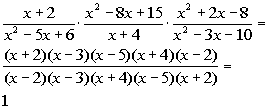*Rewrite the division as mult. of recip.

*Factor the num. and den.
*All factors divide out

Be careful.  0 is not our answer here.  When everything divides out like this, it doesn’t mean nothing is left, there is still a 1 there.Practice Problems

These are practice problems to help bring you to the next level.  It will allow you to check and see if you have an understanding of these types of problems. Math works just like anything else, if you want to get good at it, then you need to practice it.  Even the best athletes and musicians had help along the way and lots of practice, practice, practice, to get good at their sport or instrument.  In fact there is no such thing as too much practice.

To get the most out of these, you should work the problem out on your own and then check your answer by clicking on the link for the answer/discussion for that  problem.  At the link you will find the answer as well as any steps that went into finding that answer.Practice Problem 1a: Find the domain of the rational function.

1a.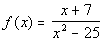Practice Problems 2a - 2c: Multiply or divide.

2a.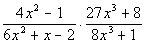2b.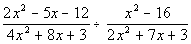2c.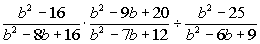Need Extra Help on these Topics?

Go to Get Help Outside the Classroom found in Tutorial 1: How to Succeed in a Math Class for some more suggestions.Last revised on July 17, 2011 by Kim Seward.